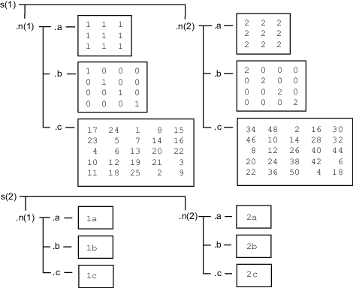Documentation

## Access Data in Nested Structures

This example shows how to index into a structure that is nested within another structure. The general syntax for accessing data in a particular field is

`structName(index).nestedStructName(index).fieldName(indices)`

When a structure is scalar (1-by-1), you do not need to include the indices to refer to the single element. For example, create a scalar structure `s`, where field `n` is a nested scalar structure with fields `a`, `b`, and `c`:

```s.n.a = ones(3); s.n.b = eye(4); s.n.c = magic(5);```

Access the third row of field `b`:

`third_row_b = s.n.b(3,:)`

Variable `third_row_b` contains the third row of `eye(4)`.

```third_row_b = 0 0 1 0```

Expand `s` so that both `s` and `n` are nonscalar (1-by-2):

```s(1).n(2).a = 2*ones(3); s(1).n(2).b = 2*eye(4); s(1).n(2).c = 2*magic(5); s(2).n(1).a = '1a'; s(2).n(2).a = '2a'; s(2).n(1).b = '1b'; s(2).n(2).b = '2b'; s(2).n(1).c = '1c'; s(2).n(2).c = '2c';```

Structure `s` now contains the data shown in the following figure.Access part of the array in field `b` of the second element in `n` within the first element of `s`:

`part_two_eye = s(1).n(2).b(1:2,1:2)`

This returns the 2-by-2 upper left corner of `2*eye(4)`:

```part_two_eye = 2 0 0 2```